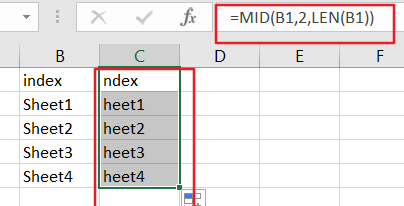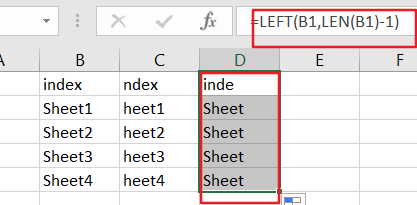# How to Extract All Characters Except the First or Last Character from Text String in Excel

This post will guide you how to extract all characters except for the first N or Last n characters from a given text string with a formula in Excel. How do I get all characters but the first or last character from a text in Excel 2013/2016.

Assuming that you have a list of data in range of Cells which contain text String values. And you wish to remove the first character or Last character or extract all characters except for the first character. You can achieve the result based on the MID function, the LEFT function and the LEN function.

## Extract All Characters Except the First Character

If you want to extract all characters except for the first character, you just need to create a new formula based on the MID function and the LEN Function. Like this:

`=MID(B1,2,LEN(B1))`

Type this formula into a blank cell or the adjacent cell C1, and press Enter key to apply it. And then drag the Auto Fill handle down to other cells to apply this formula to extract characters.Let’s see how this formula works:

The Cell B1 is the cell that you want to extract all characters but the first one character. And the number 2 is the starting position that you want to extract. And the LEN function will return the length of text string in Cell B1. So this formula can be used to extract all characters from Cell B1 except for the first character. If you want to extract all characters but the first N characters, you just need to change number 2 to N+1.

## Extract all Characters Except the Last Character

If you want to extract all characters except for the last character, you need to use a formula based on the LEFT function in combination with the LEN function. Like this:

`=LEFT(B1,LEN(B1)-1)`

Type this formula into a blank cell or cell D1, and press Enter key to apply it. And then drag the Auto Fill handle down to other cells to apply this formula to extract characters.

Note: The number 1 indicates that you want to remove the last character from the given text string in Cell B1.### Related Functions

• Excel MID function
The Excel MID function returns a substring from a text string at the position that you specify.The syntax of the MID function is as below:= MID (text, start_num, num_chars)…
• Excel LEFT function
The Excel LEFT function returns a substring (a specified number of the characters) from a text string, starting from the leftmost character.The LEFT function is a build-in function in Microsoft Excel and it is categorized as a Text Function.The syntax of the LEFT function is as below:= LEFT(text,[num_chars])…
• Excel LEN function
The Excel LEN function returns the length of a text string (the number of characters in a text string).The LEN function is a build-in function in Microsoft Excel and it is categorized as a Text Function.The syntax of the LEN function is as below:= LEN(text)…

### You might also like:

Sidebar